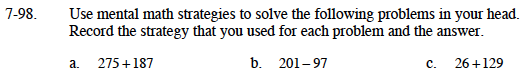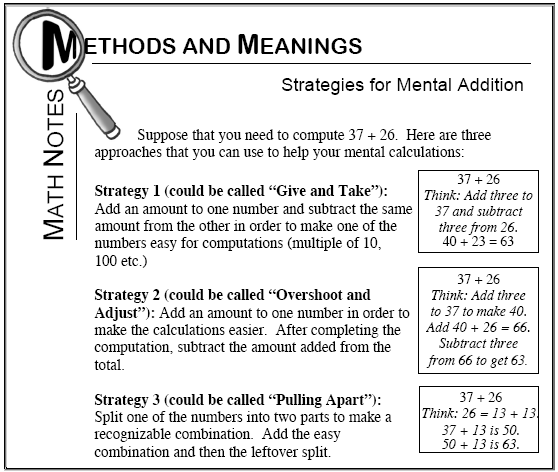### Home > MC1 > Chapter 7 > Lesson 7.2.5 > Problem7-98

7-98.The Math Notes box below describes three useful strategies. Try using ''Pulling Apart'' for this one.
You could think of 275 as 200 + 75 and 187 as 100 + 25 + 62.

You can rearrange the parts so that this sum is 200 + 100 + 75 + 25 + 62.

What is 200 − 97? Can you adjust this for 201 − 97?

Try using ''Give and Take'' for this one!

462

104